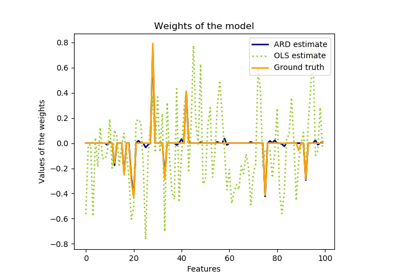# sklearn.linear_model.ARDRegression¶

class sklearn.linear_model.ARDRegression(n_iter=300, tol=0.001, alpha_1=1e-06, alpha_2=1e-06, lambda_1=1e-06, lambda_2=1e-06, compute_score=False, threshold_lambda=10000.0, fit_intercept=True, normalize=False, copy_X=True, verbose=False)[source]

Bayesian ARD regression.

Fit the weights of a regression model, using an ARD prior. The weights of the regression model are assumed to be in Gaussian distributions. Also estimate the parameters lambda (precisions of the distributions of the weights) and alpha (precision of the distribution of the noise). The estimation is done by an iterative procedures (Evidence Maximization)

Read more in the User Guide.

Parameters
n_iterint, default=300

Maximum number of iterations.

tolfloat, default=1e-3

Stop the algorithm if w has converged.

alpha_1float, default=1e-6

Hyper-parameter : shape parameter for the Gamma distribution prior over the alpha parameter.

alpha_2float, default=1e-6

Hyper-parameter : inverse scale parameter (rate parameter) for the Gamma distribution prior over the alpha parameter.

lambda_1float, default=1e-6

Hyper-parameter : shape parameter for the Gamma distribution prior over the lambda parameter.

lambda_2float, default=1e-6

Hyper-parameter : inverse scale parameter (rate parameter) for the Gamma distribution prior over the lambda parameter.

compute_scorebool, default=False

If True, compute the objective function at each step of the model.

threshold_lambdafloat, default=10 000

threshold for removing (pruning) weights with high precision from the computation.

fit_interceptbool, default=True

whether to calculate the intercept for this model. If set to false, no intercept will be used in calculations (i.e. data is expected to be centered).

normalizebool, default=False

This parameter is ignored when fit_intercept is set to False. If True, the regressors X will be normalized before regression by subtracting the mean and dividing by the l2-norm. If you wish to standardize, please use sklearn.preprocessing.StandardScaler before calling fit on an estimator with normalize=False.

copy_Xbool, default=True

If True, X will be copied; else, it may be overwritten.

verbosebool, default=False

Verbose mode when fitting the model.

Attributes
coef_array-like of shape (n_features,)

Coefficients of the regression model (mean of distribution)

alpha_float

estimated precision of the noise.

lambda_array-like of shape (n_features,)

estimated precisions of the weights.

sigma_array-like of shape (n_features, n_features)

estimated variance-covariance matrix of the weights

scores_float

if computed, value of the objective function (to be maximized)

intercept_float

Independent term in decision function. Set to 0.0 if fit_intercept = False.

Notes

For an example, see examples/linear_model/plot_ard.py.

References

D. J. C. MacKay, Bayesian nonlinear modeling for the prediction competition, ASHRAE Transactions, 1994.

R. Salakhutdinov, Lecture notes on Statistical Machine Learning, http://www.utstat.toronto.edu/~rsalakhu/sta4273/notes/Lecture2.pdf#page=15 Their beta is our self.alpha_ Their alpha is our self.lambda_ ARD is a little different than the slide: only dimensions/features for which self.lambda_ < self.threshold_lambda are kept and the rest are discarded.

Examples

>>> from sklearn import linear_model
>>> clf = linear_model.ARDRegression()
>>> clf.fit([[0,0], [1, 1], [2, 2]], [0, 1, 2])
ARDRegression()
>>> clf.predict([[1, 1]])
array([1.])


Methods

 fit(self, X, y) Fit the ARDRegression model according to the given training data and parameters. get_params(self[, deep]) Get parameters for this estimator. predict(self, X[, return_std]) Predict using the linear model. score(self, X, y[, sample_weight]) Return the coefficient of determination R^2 of the prediction. set_params(self, \*\*params) Set the parameters of this estimator.
__init__(self, n_iter=300, tol=0.001, alpha_1=1e-06, alpha_2=1e-06, lambda_1=1e-06, lambda_2=1e-06, compute_score=False, threshold_lambda=10000.0, fit_intercept=True, normalize=False, copy_X=True, verbose=False)[source]

Initialize self. See help(type(self)) for accurate signature.

fit(self, X, y)[source]

Fit the ARDRegression model according to the given training data and parameters.

Iterative procedure to maximize the evidence

Parameters
Xarray-like of shape (n_samples, n_features)

Training vector, where n_samples in the number of samples and n_features is the number of features.

yarray-like of shape (n_samples,)

Target values (integers). Will be cast to X’s dtype if necessary

Returns
selfreturns an instance of self.
get_params(self, deep=True)[source]

Get parameters for this estimator.

Parameters
deepbool, default=True

If True, will return the parameters for this estimator and contained subobjects that are estimators.

Returns
paramsmapping of string to any

Parameter names mapped to their values.

predict(self, X, return_std=False)[source]

Predict using the linear model.

In addition to the mean of the predictive distribution, also its standard deviation can be returned.

Parameters
X{array-like, sparse matrix} of shape (n_samples, n_features)

Samples.

return_stdbool, default=False

Whether to return the standard deviation of posterior prediction.

Returns
y_meanarray-like of shape (n_samples,)

Mean of predictive distribution of query points.

y_stdarray-like of shape (n_samples,)

Standard deviation of predictive distribution of query points.

score(self, X, y, sample_weight=None)[source]

Return the coefficient of determination R^2 of the prediction.

The coefficient R^2 is defined as (1 - u/v), where u is the residual sum of squares ((y_true - y_pred) ** 2).sum() and v is the total sum of squares ((y_true - y_true.mean()) ** 2).sum(). The best possible score is 1.0 and it can be negative (because the model can be arbitrarily worse). A constant model that always predicts the expected value of y, disregarding the input features, would get a R^2 score of 0.0.

Parameters
Xarray-like of shape (n_samples, n_features)

Test samples. For some estimators this may be a precomputed kernel matrix or a list of generic objects instead, shape = (n_samples, n_samples_fitted), where n_samples_fitted is the number of samples used in the fitting for the estimator.

yarray-like of shape (n_samples,) or (n_samples, n_outputs)

True values for X.

sample_weightarray-like of shape (n_samples,), default=None

Sample weights.

Returns
scorefloat

R^2 of self.predict(X) wrt. y.

Notes

The R2 score used when calling score on a regressor will use multioutput='uniform_average' from version 0.23 to keep consistent with r2_score. This will influence the score method of all the multioutput regressors (except for MultiOutputRegressor). To specify the default value manually and avoid the warning, please either call r2_score directly or make a custom scorer with make_scorer (the built-in scorer 'r2' uses multioutput='uniform_average').

set_params(self, **params)[source]

Set the parameters of this estimator.

The method works on simple estimators as well as on nested objects (such as pipelines). The latter have parameters of the form <component>__<parameter> so that it’s possible to update each component of a nested object.

Parameters
**paramsdict

Estimator parameters.

Returns
selfobject

Estimator instance.

## Examples using sklearn.linear_model.ARDRegression¶